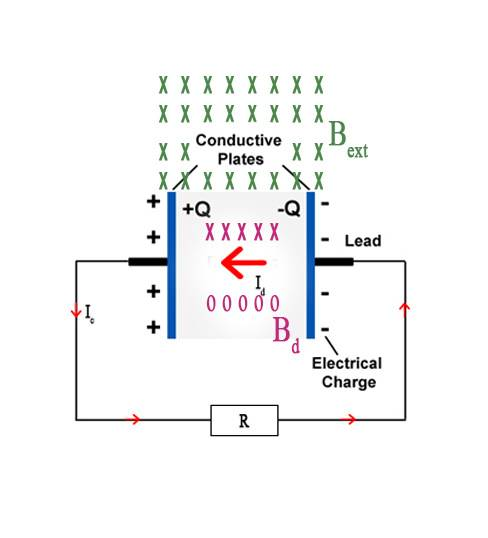# Discharging a capacitor faster using an external B-field?

PhiowPhi
If a capacitor is being discharged and the electric field is varying with time producing the displacement currents, wouldn't it be possible to align a time-varying external magnetic field with the magnetic field produced by the displacement currents to increase the discharge rate? Or the vice-versa slow down the discharge rate?$$B_{Net} = \Delta B_d + \Delta B_{ext} ∴ \uparrow t_d$$
$$B_{Net} = \Delta B_d - \Delta B_{ext} ∴ \downarrow t_d$$

Where,
##t_d##: The discharge time.
##B_{Net}## : The net magnetic field within the separation region.

From Ampere's law it seems plausible:

$$\oint_C {Bd\ell = \mu _0 I_C }$$

#### Attachments

•Nik_2213

## Answers and Replies

Gold Member
The time varying magnetic field can encompass the space between the capacitor plates or the connecting wires with equal effect. It will induce a voltage in series with the circuit by electromagnetic induction, and is equivalent to placing a dynamo in series with the circuit. If the polarity is such as to aid the flow of current, the capacitor will discharge quicker.
The energy supplied by the dynamo will be expended in additional heating of R.

•anorlunda
Nik_2213
#PP: Slightly tangential but, given many capacitors are 'rolled up' for volumetric efficiency, and air-gapped variable capacitors are usually multi-plate, so the effects of an external magnetic field would cancel, you'll have difficulties applying this tweak.
Kudos for the lateral thinking !!

•sophiecentaur
Gold Member
Is this a thought experiment or is there a practical aspect to it. Is the aim to discharge the capacitor as fast as possible or is it to pass a high current through the R?
I'm sort of looking for a Switch somewhere in the circuit??
The current through the load R will depend on the voltage across it.

2021 Award
The need to discharge a capacitor rapidly is usually encountered when resetting a charge integrator circuit. Unfortunately the current in the inductance of the coil needed to generate the magnetic field takes longer to build than does short circuiting the capacitor, and then you must wonder what will happen when you try to turn off the magnetic field.

To discharge 1 volt, from a 1 farad capacitor, in 1 second, would only need a current of one amp. To discharge it in 1 nanosecond would require an impossible Giga-amp. By employing commutation, a charge integrator of any capacitance, can be used to generate a sawtooth waveform with a one nanosecond transition time.

Why do you need to discharge the capacitor so quickly?